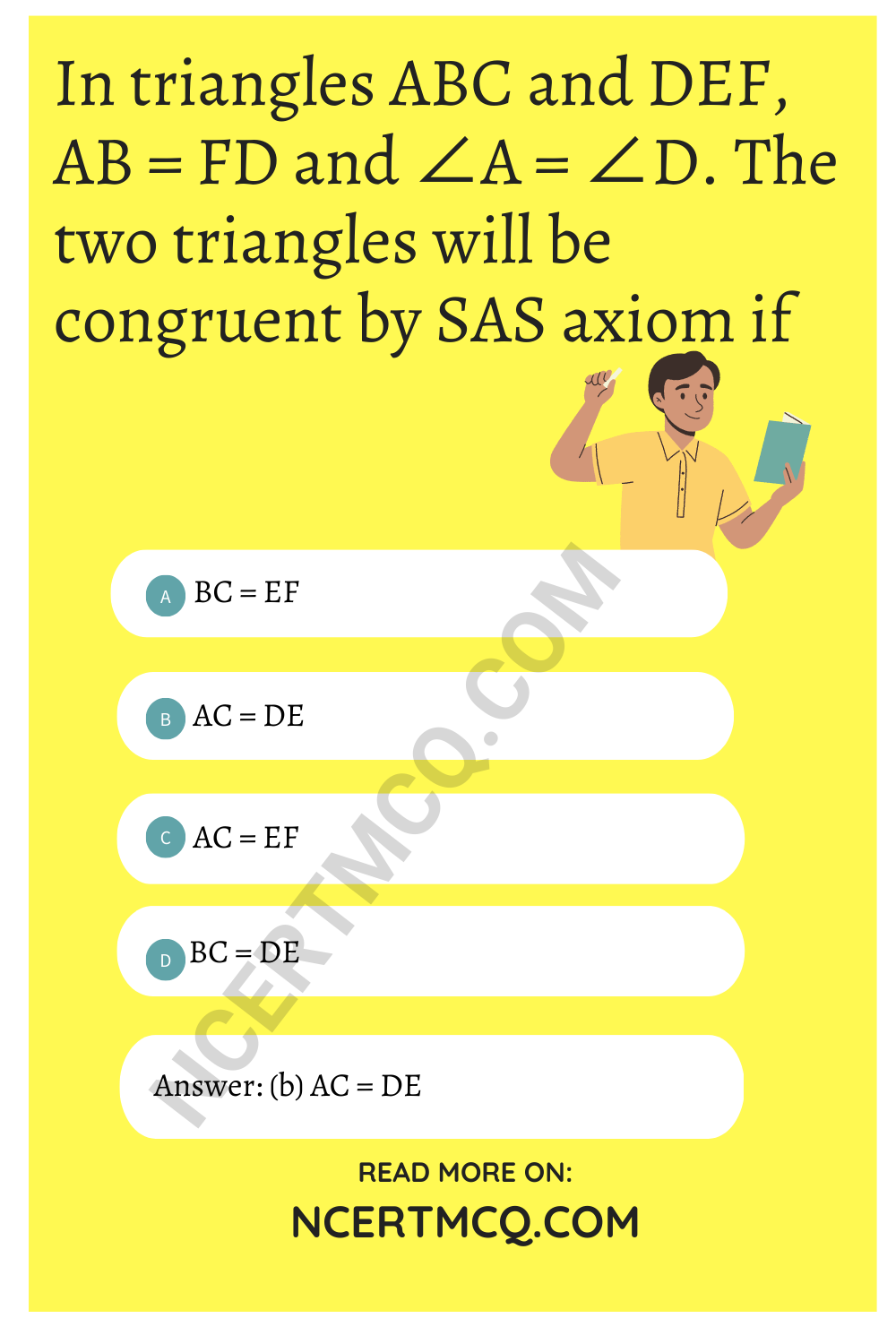Check the below Online Education NCERT MCQ Questions for Class 9 Maths Chapter 7 Triangles with Answers Pdf free download. MCQ Questions for Class 9 Maths with Answers were prepared based on the latest exam pattern. We have provided Triangles Class 10 Maths MCQs Questions with Answers to help students understand the concept very well. https://ncertmcq.com/mcq-questions-for-class-9-maths-with-answers/

Students can also refer to NCERT Solutions for Class 9 Maths Chapter 7 Triangles for better exam preparation and score more marks.

## Triangles Class 9 MCQs Questions with Answers

Triangles Class 9 MCQ Chapter 7 Question 1.
In given figure, the measure of ∠BAC is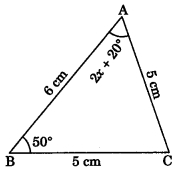(a) 60°
(b) 50°
(c) 70°
(d) 80°

Triangles Class 9 MCQ With Answers Chapter 7 Question 2.
In figure if AE || DC and AB = AC, find the value of ∠ABD.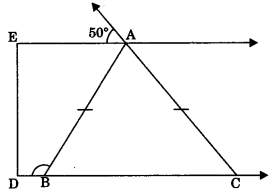(a) 130°
(b) 110°
(c) 120°
(d) 70°

Triangles MCQ Class 9 Chapter 7  Question 3.
In figure X is a point in the interior of square ABCD, AXYZ is also a square. If DY = 3 cm and AZ = 2 cm, then find BY.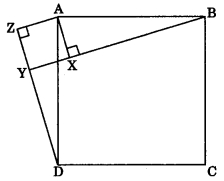(a) 5 cm
(b) 6 cm
(c) 7 cm
(d) 8 cm

Class 9 Triangles MCQ Chapter 7 Question 4.
In figure AB ⊥ AE, BC ⊥ AB, CE = DE and ∠AED = 120°, then find ∠ECD.(a) 80°
(b) 70°
(c) 85°
(d) 60°

Class 9 Maths Chapter 7 MCQ Question 5.
In ΔABC, ∠C = ∠A and BC = 4 cm and AC = 5 cm, then find length of AB.
(a) 5 cm
(b) 3 cm
(c) 4 cm
(d) 2.5 cm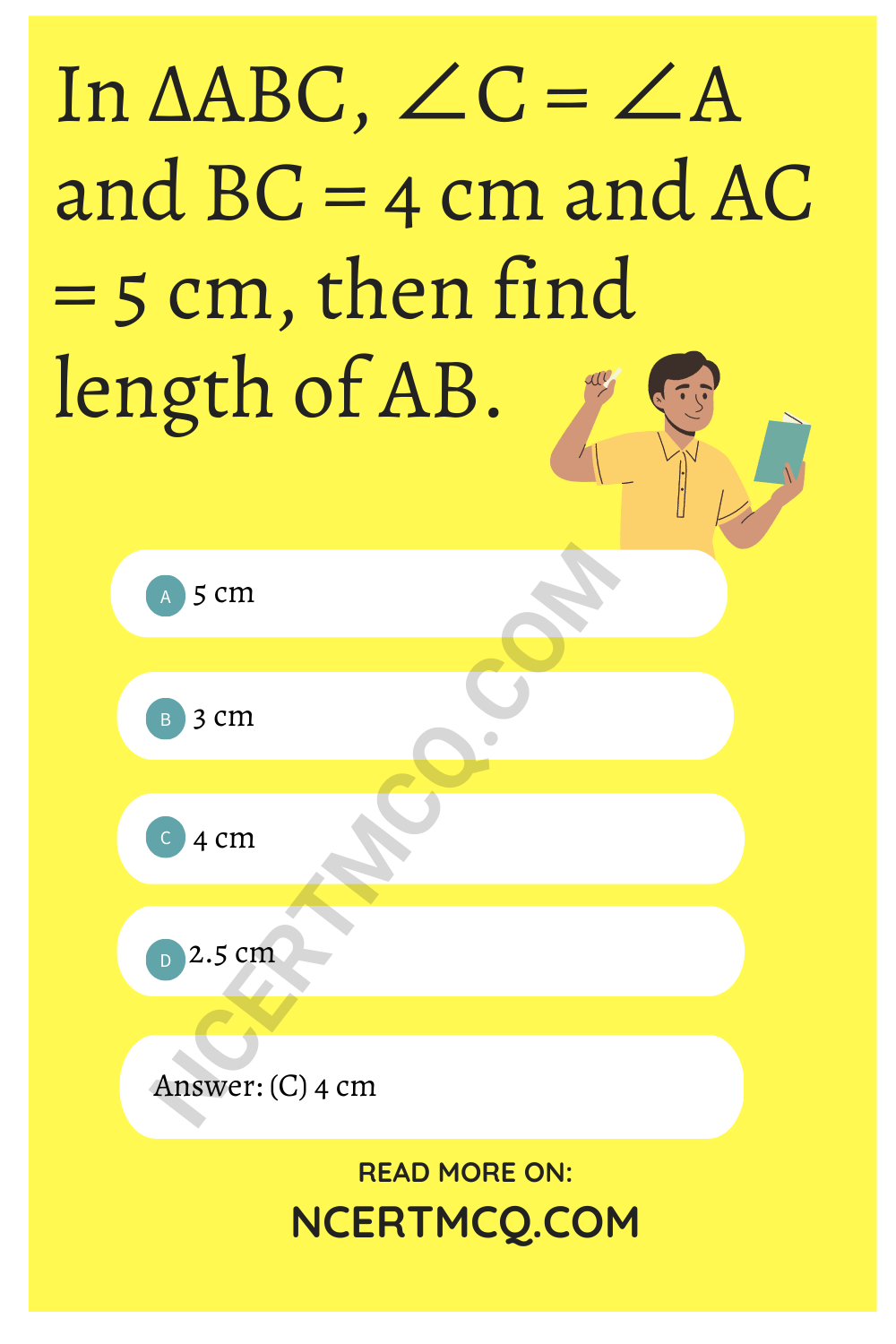Triangle Class 9 MCQ Chapter 7 Question 6.
In ΔABC, AB = AC and ∠B = 50°, then find ∠C.
(a) 50°
(b) 40°
(c) 80°
(d) 120°

Triangle MCQ Class 9 Chapter 7 Question 7.
In figure, D is the mid-point of side BC of a ΔABC and ∠ABD = 50°. If AD = BD = CD, then find the measure of ∠ACD.(a) 30°
(b) 70°
(c) 80°
(d) 40°

MCQ On Triangles Class 9 Chapter 7 Question 8.
In figure, ABC is an isosceles triangle whose side AC is produce to E and through C, CD is drawn parallel to BA. Find the value of x.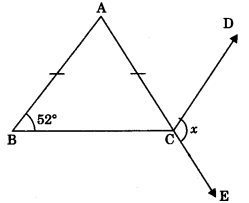(a) 52°
(b) 156°
(c) 76°
(d) 104°

MCQ Of Triangles Class 9 Chapter 7 Question 9.
In figure AB ⊥ BE and EF ⊥ BE. If BC = DE and AB = EF, then ΔABD is congruent to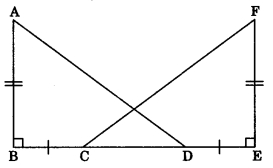(a) ΔEFC
(b) ΔECF
(c) ΔDEF
(d) ΔFEC

Ch 7 Maths Class 9 MCQ Chapter 7 Question 10.
D is a point on the side BC of a ΔABC such that AD bisects ∠BAC. Then
(a) BD = CD
(b) BA > BD
(c) BD > BA
(d) CD > CA

Class 9 Maths Ch 7 MCQ Question 11.
It is given that ΔABC = ΔFDE and AB = 5 cm, ∠B = 40° and ∠A = 80°. Then which of the following is true?
(а) DF = 5 cm, ∠F = 60°
(b) DF = 5 cm, ∠E = 60°
(c) DE = 5 cm, ∠E = 60°
(d) DE = 5 cm, ∠D = 40°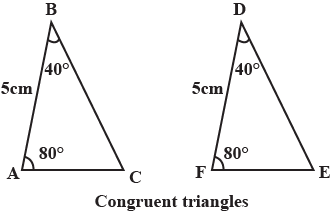Answer: (b) DF = 5 cm, ∠E = 60°

Two triangles are congruent if their corresponding sides are equal in length and their corresponding angles are equal in size.
In the first option, DF = 5 cm but ∠F is not equal to 60°.
In the third and the fourth options, the length of DE ≠ 5cm, since it is not defined.
In the second option, DF = 5cm and ∠E = 60° is satisfied.

Class 9 Maths Triangles MCQ Chapter 7 Question 12.
Two sides of a triangle are of lengths 5 cm and 1.5 cm. The length of the third side of the triangle cannot be
(a) 3.6 cm
(b) 4.1 cm
(c) 3.8 cm
(d) 3.4 cm

Chapter 7 Maths Class 9 MCQ Question 13.
In ΔPQR, if ∠R > ∠Q, then
(a) QR > PR
(b) PQ > PR
(c) PQ < PR
(d) QR < PR

Triangles Class 9 MCQ With Answers Pdf Question 14.
In triangles ABC and PQR, AB = AC, ∠C = ∠P and ∠B = ∠Q. The two triangles are
(a) isosceles but not congruent
(b) isosceles and congruent
(c) congruent but not isosceles
(d) neither congruent nor isosceles

Answer: (a) isosceles but not congruent

MCQ Triangles Class 9 Chapter 7 Question 15.
In triangles ABC and DEF, AB = FD and ∠A = ∠D. The two triangles will be congruent by SAS axiom if
(a) BC = EF
(b) AC = DE
(c) AC = EF
(d) BC = DE